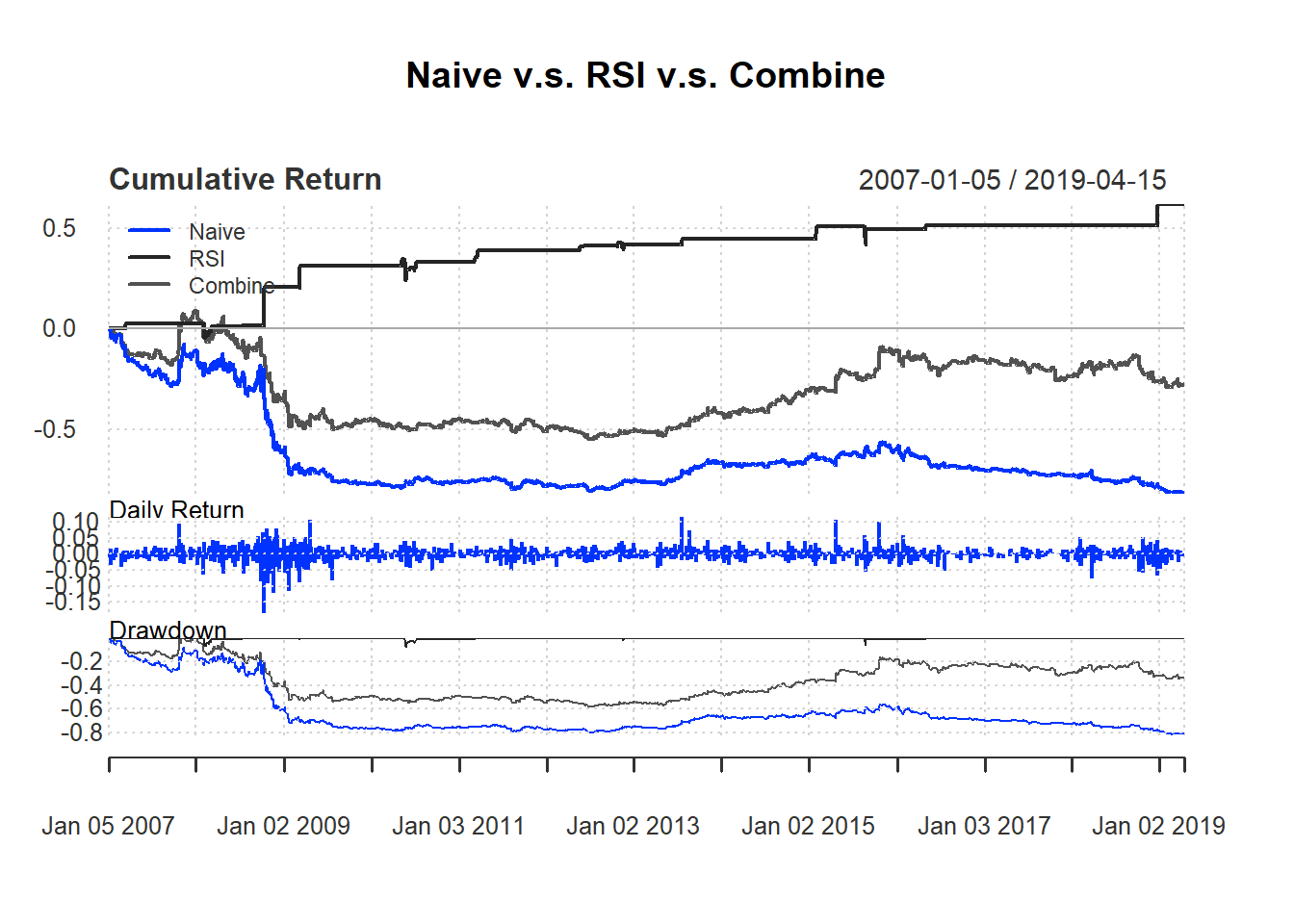## 7.8 Combining two indicators: EMA and RSI

Test the following strategy using EMA and RSI based on day trading:

• Buy signal based on EMA rule.

• Sell signal based on RSI rule.

• We use 14-day RSI and use 70 as threshold for selling.

n <- 14
delta<-0.005
price <- Cl(MSFT)
r <- price/Lag(price) - 1
rsi <- RSI(price, n)
signal <-c()    # first signal is NA
signal[1:n] <-0

for (i in (n+1):length(price)){
if (r[i] > delta){
signal[i]<- 1
} else if (rsi[i] > 70){
signal[i]<- -1
} else
signal[i]<- 0
}
signal<-reclass(signal,price)

names(ret3) <- 'Combine'
retall <- cbind(ret1, ret2, ret3)

To draw trade performance summary with different colors, we use the option colorset. Common options includes redfocus, bluefocus, greenfocus, rainbow4equal andrich12equal.

charts.PerformanceSummary(
retall, main="Naive v.s. RSI v.s. Combine",
colorset=bluefocus)### Exercise

Test the following strategy based on EMA and RSI

• Buy signal based on EMA rule.

• Sell signal based on RSI rule

• Day trading based on yesterday signal: buy at open and sell at close